# Pair of Linear Equations in Two Variables Class 10 Notes Chapter 3

## CBSE Class 10 Maths Chapter 3 Pair Of Linear Equations In Two Variables Notes

CBSE Class 10 Maths Chapter 3 Pairs of Linear Equations in Two Variables notes are available here. This article is provided here for students of Class 10 to have quick revision for the chapter “Pairs of Linear Equations in Two Variables”. This chapter will help you to determine the values of the unknown variables with the help of various methods, such as the Graphical method and Algebraic methods. An example of pairs of linear equations will be: Rohan bought 2 pencils and 1 pen for Rs.10, whereas Sam bought 4 pencils and 2 pens for Rs. 20, so what is the price of each pen and pencil? Now to find the solution to this question, we can take pen and pencil as two different variables and write the linear equations for each condition. Hence, by solving the pair of linear equations in two variables, we will get the price of a pen and pencil. Let us go through the article to understand how to solve such a pair of equations.

## Basics Revisited

### Equation

An equation is a statement that two mathematical expressions having one or more variables are equal.

### Linear Equation

Equations in which the powers of all the variables involved are one are called linear equations. The degree of a linear equation is always one.

To know more about Linear Equations, visit here.

## Video Lesson on Pair of Linear Equations in Two Variables### General Form of a Linear Equation in Two Variables

The general form of a linear equation in two variables is ax + by + c = 0, where a and b cannot be zero simultaneously.

Students can refer to the short notes and MCQ questions along with separate solution pdf of this chapter for quick revision from the links below:

### Representing Linear Equations for a Word Problem

To represent a word problem as a linear equation:

• Identify unknown quantities and denote them by variables.
• Represent the relationships between quantities in a mathematical form, replacing the unknowns with variables.

Example: The cost of 5 pens and 7 pencils is Rs.50 whereas the cost of 7 pens and 5 pencils is Rs. 65. Represent the given word problem in linear equations form.

Solution: Let us say the cost of 1 pen is Rs.x, and the cost of 1 pencil is Rs.y
Given,
The cost of 5 pens and 7 pencils is Rs.50.
So, we can write in the form of a linear equation;
5x + 7y = 50
Again given,
The cost of 7 pens and 5 pencils is Rs.65
So, we can write in the form of a linear equation;
7x + 5y = 65
Hence, 5x + 7y = 50 and 7x + 5y = 65 are the pairs of linear equations in two variables as per the given word problem.

### Solution of a Linear Equation in 2 Variables

The solution of a linear equation in two variables is a pair of values (x, y), one for ‘x’ and the other for ‘y’, which makes the two sides of the equation equal.
Eg: If 2x + y = 4, then (0,4) is one of its solutions as it satisfies the equation. A linear equation in two variables has infinitely many solutions.

### Geometrical Representation of a Linear Equation

Geometrically, a linear equation in two variables can be represented as a straight line.
2x – y + 1 = 0
⇒ y = 2x + 1
Let us draw the graph for the given equation.

Step 1: Put the value of x = 0, such that y = 1.

Step 2: Put the value of x = -1/2 or 0.5, such that y = 0

Hence, the coordinates are (0, 1) and (-0.5, 0). We can find more points to plot the graph by putting different values for x. Now based on these coordinates, we can draw a straight line in the graph connecting the two points (0, 1) and (-0.5, 0) as shown in the figure below.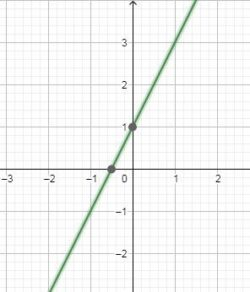To know more about Linear Equations in Two Variables, visit here.

### Plotting a Straight Line

The graph of a linear equation in two variables is a straight line. We plot the straight line as follows: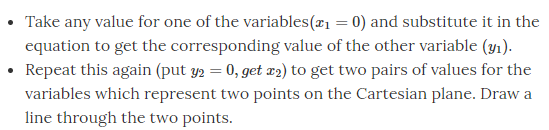Any additional points plotted in this manner will lie on the same line.

For example, in the above section, we have plotted the graph for y = 2x + 1, which shows a straight line passing through the points (0, 1) and (-0.5, 0).

Know More: Graphing of Linear Equations

## General Form of a Pair of Linear Equations in 2 Variables

A pair of linear equations in two variables can be represented as follows: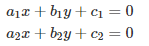The coefficients of x and y cannot be zero simultaneously for an equation.

### Nature of 2 Straight Lines in a Plane

For a pair of straight lines on a plane, there are three possibilities.

i) They intersect at exactly one point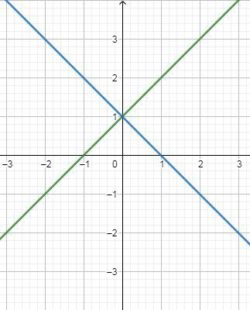ii) They are parallel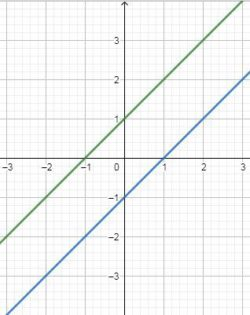iii) They are coincident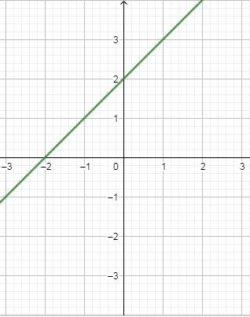## Graphical Solution

### Representing Pair of LE in 2 Variables Graphically

Graphically, a pair of linear equations in two variables can be represented by a pair of straight lines.

### Graphical Method of Finding Solution of a Pair of Linear Equations

The Graphical Method of finding the solution to a pair of linear equations is as follows:

• Plot both the equations (two straight lines)
• Find the point of intersection of the lines.

The point of intersection is the solution.
For example, the graph of two linear equations 2x + y – 6 = 0 and 4x – 2y – 4 = 0 is shown below. The point of intersection for the two graphs is (2,2).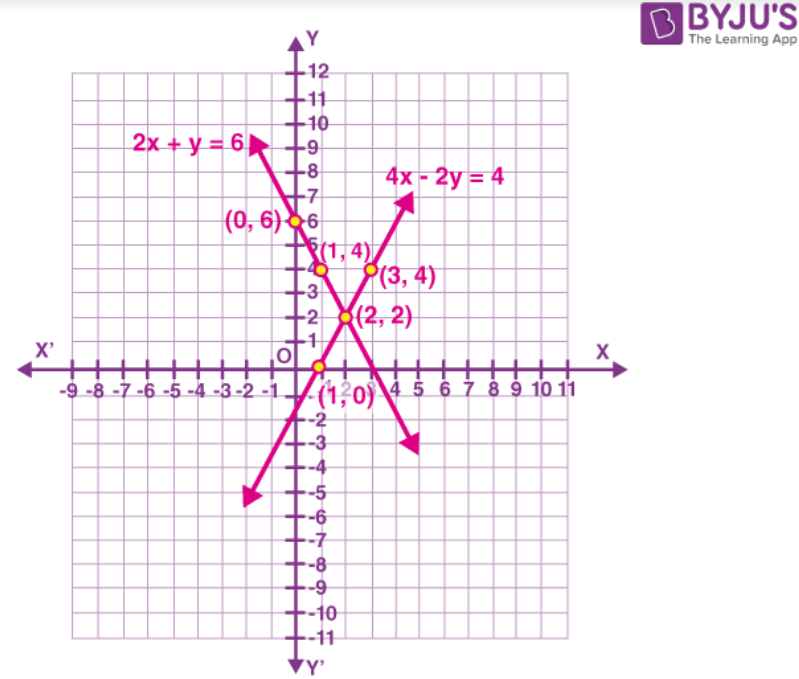To know more about Graphing of Linear Equations in 2 Variables, visit here.

###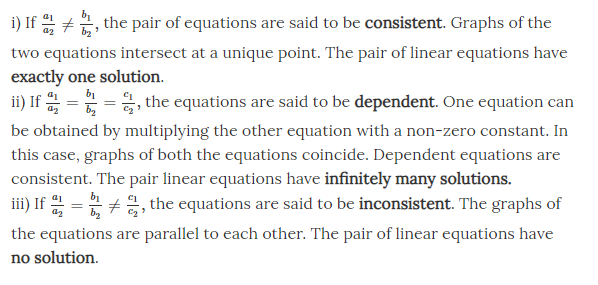Example: On comparing the ratio, (a1/a2) , (b1/b2) , (c1/c2) find out whether 2x – 3y = 8 and 4x – 6y = 9 are consistent, or inconsistent.

Solution: As per the given pair of linear equations, 2x – 3y = 8 and 4x – 6y = 9, we have;

a1 = 2, b1 = -3, c1 = -8

a2 = 4, b2 = -6, c2 = -9

(a1/a2) = 2/4 = 1/2

(b1/b2) = -3/-6 = 1/2

(c1/c2) = -8/-9 = 8/9

Since , (a1/a2) = (b1/b2) ≠ (c1/c2)

So, the given linear equations are parallel to each other and have no possible solution. Hence, the given pair of linear equations is inconsistent.

#### For more information on Finding Solutions for Consistent and Inconsistent Pairs of Linear Equations, watch the below video## Algebraic Solution

### Finding Solutions for Consistent Pairs of Linear Equations

The solution of a pair of linear equations is of the form (x,y), which satisfies both equations simultaneously. Solution for a consistent pair of linear equations can be found using

i) Elimination method

ii) Substitution Method

iii) Cross-multiplication method

iv) Graphical method

To know more about Finding the Solution of Algebraic Expressions, visit here.

### Substitution Method of Finding Solution of a Pair of Linear Equations

Substitution method:

y – 2x = 1

x + 2y = 12

(i) express one variable in terms of the other using one of the equations. In this case, y = 2x + 1.

(ii) substitute for this variable (y) in the second equation to get a linear equation in one variable, x. x + 2 × (2x + 1) = 12

⇒ 5 x + 2 = 12

(iii) Solve the linear equation in one variable to find the value of that variable.
5 x + 2 = 12
x = 2

(iv) Substitute this value in one of the equations to get the value of the other variable.

y = 2 × 2 + 1

⇒y = 5

So, (2,5) is the required solution of the pair of linear equations y – 2x = 1 and x + 2y = 12.

### Elimination Method of Finding Solution of a Pair of Linear Equations

Elimination method
Consider x + 2y = 8 and 2x – 3y = 2

Step 1: Make the coefficients of any variable the same by multiplying the equations with constants. Multiplying the first equation by 2, we get,

2x + 4y = 16

Step 2: Add or subtract the equations to eliminate one variable, giving a single variable equation.
Subtract the second equation from the previous equation
2x + 4y = 16
2x  – 3y =  2
–     +       –
———————–
0(x) + 7y =14
Step 3: Solve for one variable and substitute this in any equation to get the other variable.

y = 2,

x = 8 – 2 y

⇒ x = 8 – 4

⇒ x = 4

(4, 2) is the solution.

### Cross-Multiplication Method of Finding Solution of a Pair of Linear Equations

For the pair of linear equations

a1+ b1+ c1=0

a2x + b2y + c2=0,
x and y can be calculated as

x = (b1c2−b2c1)/(a1b2−a2b1)

y = (c1a2−c2a1)/(a1b2−a2b1)

## Equations Reducible to a Pair of Linear Equations in 2 Variables

In this section, we learn about such equations, which are not linear but can be reduced to a pair of linear equations form using the substitution method. Let us understand with the help of an example.

2/x+3/y=4

5/x−4/y=9

In this case, we may make the substitution

1/x = u and 1/y = v

The pair of equations reduces to
2u + 3v = 4 …..(i)
5u – 4v = 9 ….(ii)
From the first equation, isolate the value of u.
u = (4-3v)/2

Now substitute the value of u in eq. (ii)
5[(4-3v)/2] – 4v = 9
Solving for v, we get;
v = 2/23
Now substitute the value of v in u = (4-3v)/2, to get the value of u.
u = 43/23

Since, u = 1/x or x = 1/u = 23/43

and v = 1/y or y = 1/v, so y = 23/2

Hence, the solutions are x = 23/43 and y = 23/2.

## Frequently Asked Questions on CBSE Class 10 Maths Notes Chapter 3: Pair of Linear Equations in Two Variables

Q1

### What is a linear equation?

A linear equation is an equation in which the highest power of the variable is always 1.

Q2

### What is an algebraic equation?

An algebraic equation can be defined as a mathematical statement in which two expressions are set equal to each other.

Q3

### What are some of the uses of linear equations?

1. Solve age-related problems 2. Calculate speed 3. Geometry-related problems 4. Work, time and wage-related problems 5. Calculate money, percentage-related sums